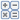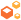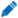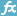# Configure a Formula on an eForm

To configure a formula on an eForm, do the procedure in this topic.

## How to Start

1. On the Application Explorer screen, do one of these:
2. Do one of these:
• Add a Formulacontrol:
1. On the eForm Builder screen, in the Toolbox, open the Advanced Controlstab.
2. On the Advanced Controlstab, drag a Formulaform control onto your eForm.
• Change a Formulacontrol:
1. On your eForm, click the control, and click Edit.
3. Complete the fields as necessary.
4. On the eForm Builder screen, click the Formulaform control, and click Formula.

## Procedure

1. On the Formula Builder screen, in the Fields/Tokens list, select the form control to create a formula.
2. In the Operators list, select the operator to compare the values in your logical expression.
Field Name Definition

Operator

Function:
Specifies the operator to use in the formula.
Accepted Values:
• +
• -
• *
• /
• %
• <=
• <
• >=
• >
• !=
• =
• &&
• ||
Default Value:
None
Example:
Refer to:
3. (Optional) In the Keywords field, select the keyword to use in your formula.
Field Name Definition

Keywords

Function:
Shows the list of keywords to use in the formula to show specific values on the eForm.
Accepted Values:
• randomstring - Let's you use a random alphanumeric string on the eForm which can be used to validate the form user.
• now - Let's you use the current day in the formula.
• days - Let's you use the current day as a number. For example, Sunday is 0, Monday is 1 and so on.
• months - Let's you use the month in the formula.
• years- Let's you use the year in the formula.
Default Value:
• randomstring
• now
• days
• months
• years
4. In the Function field, select the function to use in your formula.
Field Name Definition

Function

Function:
Shows the list of functions to use in the formula to do specific actions on the eForm.
Accepted Values:
• datediff - Gets the difference in hours, minutes, seconds, days, weeks, or months between two dates.
• sum - Gives the sum of a group of numbers.
• dow - Gets the number of week days from a specified date.
• instr - Searches a string for a substring using characters and returns the position in the string that is the first character of a specified occurrence of the substring.
• lowerstr - Changes the capital letters of a specified string expression to small letters.
• upperstr - Changes the small letters of a specified string expression to capital letters.
• substr - Gives the characters in a string that starts at the specified location, and ends at the specified number of characters.
• if - Specifies an if condition.
• decimal - Gives a number in decimal format.
Default Value:
None
Example:
Refer to:
5. Click Insert to add the function into the formula.
6. To make sure the formula is correct, click Validate.